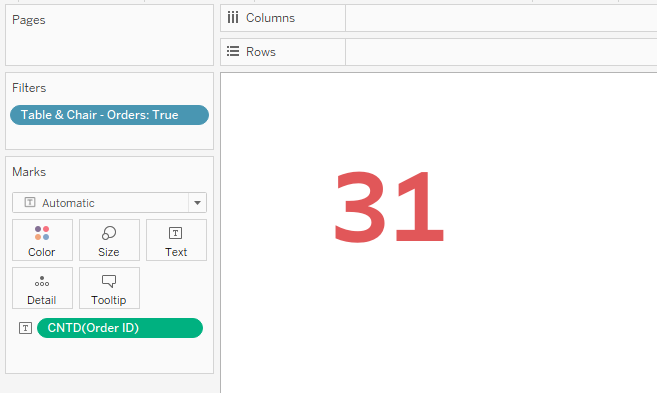top of page
Search
• Bernard K

# Combining IF statement with FIXED LOD in Tableau

Updated: Nov 7, 2022### Introduction

IF statement tests an expression, returning one variable if the expression is TRUE and another variable is the expression is FALSE or OTHERWISE.

FIXED LOD on the other hand is an expression that computes values on a specific dimension without reference to any other dimensions present in the view.

In this short article, I am going to explore one use case in which these two expressions can be used to answer a business question.

### Use Case: Using Sample-Superstore data, find the number of orders that contain both Tables and Chairs?

(Note, the Sample-Superstore data used in this article might differ to some extent – so don’t worry incase you get different results).

To answer this question, create a calculation which returns TRUE for orders that contain both Tables and Chairs using the calculation below.Notice when you add this calculation to the view below - returns TRUE for orders that contain ‘Tables’ and ‘Chairs’.If you need to see ONLY orders that contain both Tables and Chairs, drag the calculation ‘Table & Chairs – Orders’ to the filter shelf and select ‘TRUE’.With this calculation - you can compute the number of orders that contain both the Tables and Chairs as follows.

• Drag COUNTD(Order-ID) to the text/label shelf (which returns all the unique orders)

• Drag the calculation ‘Table & Chairs – Orders’ to the filter shelf and select ‘TRUE’Which returns the total orders that contain both Chairs and Tables as 31.

If you like the work we do and would like to work with us, drop us an email on our Contacts page and we’ll reach out!